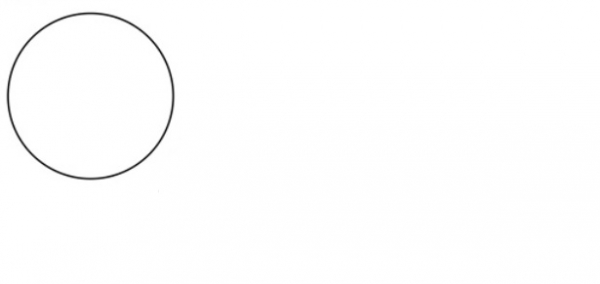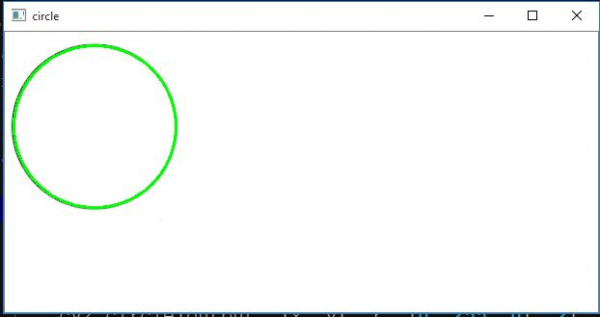# Find Circles in an Image using OpenCV in Python

The OpenCV platform provides cv2 library for python. This can be used for various shape analysis which is useful in computer vision. In this article we will identify the shape of a circle using Open CV. For that we will use the cv2.HoughCircles() function.Finds circles in a grayscale image using the Hough transform. In the below example we will take an image as input. Then make a copy of it and apply this transform function to identify the circle in the output.

## Syntax

cv2.HoughCircles(image, method, dp, minDist)
Where
Image is the image file converted to grey scale
Method is the algorithm used to detct the circles.
Dp is the inverse ratio of the accumulator resolution to the image resolution.
minDist is the Minimum distance between the center coordinates of detected circles.

## Example

In the below example we use the image below as our input image. Then run the below program to get the circles.The below program detects the presence of circle in an image file. If circle is present then it highlights it.

## Example

import cv2
import numpy as np
output = image.copy()
img = cv2.cvtColor(image, cv2.COLOR_BGR2GRAY)
# Find circles
circles = cv2.HoughCircles(img, cv2.HOUGH_GRADIENT, 1.3, 100)
# If some circle is found
if circles is not None:
# Get the (x, y, r) as integers
circles = np.round(circles[0, :]).astype("int")
print(circles)
# loop over the circles
for (x, y, r) in circles:
cv2.circle(output, (x, y), r, (0, 255, 0), 2)
# show the output image
cv2.imshow("circle",output)
cv2.waitKey(0)

Running the above code gives us the following result −

## Output

[[93 98 84]]

And we get the below diagram showing the output.